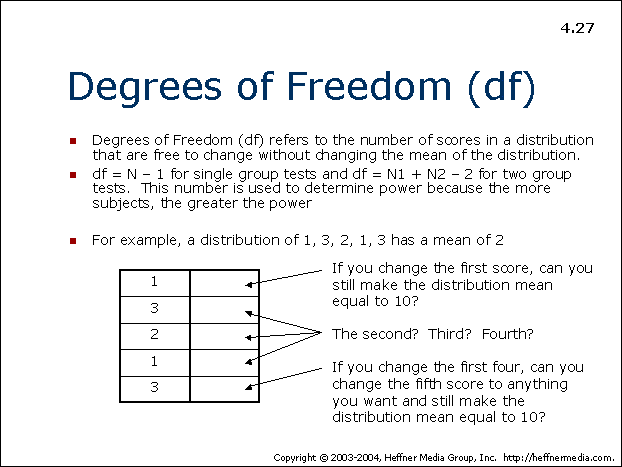# 27: Degrees of Freedom (df)## Degrees of Freedom (df)

• Degrees of Freedom (df) refers to the number of scores in a distribution that are free to change without changing the mean of the distribution.
• df = N – 1 for single group tests and df = N1 + N2 – 2 for two group tests. This number is used to determine power because the more subjects, the greater the power
• For example, a distribution of 1, 3, 2, 1, 3 has a mean of 2

If you change the first score, can you still make the distribution mean equal to 10?

The second? Third? Fourth?

If you change the first four, can you change the fifth score to anything you want and still make the distribution mean equal to 10?